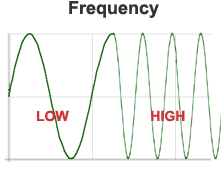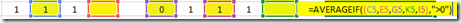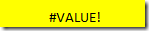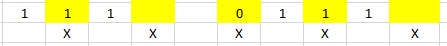# How-to AverageIf Excluding Zeros and Blanks for Non-Contiguous Ranges in ExcelIn our last Friday Challenge, I asked you figure out a way to calculate an average for a non-contiguous (non-adjacent) range that will exclude blanks and zeros.

This came from a user question on a previous post:

Excel Formulas: Average a Range and Ignore Zero’s (0 Value)

where they asked how to do this for non-adjacent columns of data.If you try and use the AverageIf function above, you will get a #Value ErrorIt appears that AverageIf doesn’t work on a non-contiguous range.  So how can we do this?

Version 1:

Ken detailed using AverageIfs formula.  Here is how he did it.

1) Add an “X” in a helper row of data so that we can use these in the AverageIfs calculation to determine the non-contiguous data range:2) Next, we want to create our AverageIfs formula as such:

=AVERAGEIFS(B5:K5,B5:K5,”>0″,B6:K6,”X”)

Here is a breakdown of the formula:

a) B5:K5 as the average range is used for the values in the average calculation

b) B5:K5,”>0” checks to see if the value in the data is greater than zero and therefore include it in the calculation.  Blanks are automatically excluded as they are not numbers.

c) B6:K6,”X” checks to if there is an X and therefore include that column of data in the average calculation

# If you found the website and tutorials helpful, please consider donating to keep the lights on.

## Donate with PayPal here:Note: If you copy and paste this solution, you may have to delete and re-input the “ quotation marks as they may not be the correct quote version for your Excel version.

Note: This solution doesn’t work if you have a negative value in your non-contiguous data range.  Can you figure it out?  If you figure it out, post a comment below with your solution.

Version 2:

1) Create the following formula to calculate your own average.  Averages are just a sum of the total amount divided by the count of values.  This formula does just that.

=SUM(C3,E3,G3,I3,K3)/INDEX(FREQUENCY((C3,E3,G3,I3,K3),0),2)

Here is a breakdown of the formula:

a) =SUM(C3,E3,G3,I3,K3)/ – this is the Numerator and is simply adding the non-contiguous range in a SUM function.  SUM doesn’t have a problem with non-contiguous ranges.

b) INDEX(FREQUENCY((C3,E3,G3,I3,K3),0),2) – this is the Denominator and is a combination of Index and Frequency

b1) FREQUENCY((C3,E3,G3,I3,K3),0) – since frequency can use non-contiguous ranges, we will use this to count the number of times that we have a zero in the data and the number of times that the data is not equal to zero.  The result of this formula will be an array of 2 numbers {Count of Zeros and Blank Values; Count of Greater than Zero Values} – i.e. {1,4}

b2) INDEX({1,4},2) – Since the frequency function we created will return 1 of 2 numbers, we only want to return one of these values as our count for the denominator.  In our case, we have an array of data before the comma and we use the 2 in the value after the comma to show that we want to return the 2nd value (count of greater than zero values).

Video Demonstration

Solutions – Average Excluding Zeros and Blanks for Non-Adjacent Columns

What other use cases do you see for Frequency?  The first one that comes to mind is for a histogram graph, but what are other ideas for this function?

Steve=True

1.Leonid

This one should do the trick:
=SUM(C2,E2,G2,I2,K2)/COUNT((1/CHOOSE(COLUMN(\$1:\$1),C2,E2,G2,I2,K2)))

2.Ken G

=AVERAGEIFS(B5:K5,B5:K5,”>0″,B6:K6,”X”)

simply change the >0 to (not equal) 0

=AVERAGEIFS(B5:K5,B5:K5,”0″,B6:K6,”X”)

•SteveEqualsTrue

Thanks Ken. Nice solution. I think your formula may have been typed in wrong or the browser dropped some information as it was missing the <> not equals operators. =AVERAGEIFS(B5:K5,B5:K5,”<>0″,B6:K6,”X”). Thanks again. Steve=True

• # If you found the website and tutorials helpful, please consider donating to keep the lights on.

## Donate with PayPal here:•Ms. M.

Hi Steve, your video was extremely helpful. I am currently stuck with one issue, the formula shows up with either #VALUE! or #DIV/0! when the cells are blank. I do want to include zeros. Hope you can help me. Thank you, M.

=AVERAGE(IF(E\$4:E\$52,E\$4:E\$52,”0″),\$B\$4:\$B\$52,”Name”)
=AVERAGEIFS(E\$4:E\$52,E\$4:E\$52,”0″,\$B\$4:\$B\$52,”Name”)

•SteveEqualsTrue

Hi Ms M. thanks for your great comment. Since you want to ignore blanks but include zeros, wouldn’t a simpler formula work? =AVERAGEIF(E\$4:E\$52,\$B\$4:\$B\$52,”Name”) Hope this helps. Steve=True

•Melissa House

This is great! But how do you do this over multiple sheets? Same cells in each sheet (C10, C27, C43) over 24 sheets.

•SteveEqualsTrue

Hi Melissa,

I have not tried for this example specifically, but you can average across sheets like this:
=AVERAGE(Sheet1!A3,Sheet2!A3,Sheet3!A3)

Let me know if that helps.

Steve=True

•Erian

hello, I tried to use the formula but it is not averaging for me. It is adding it for me. Why is that?

•SteveEqualsTrue

Hi Erian, I would have to see what formula you used. However, I would recommend if you copy/pasted from the website to replace your ” (quotes) in the formula by retyping them. Web versions of the quotes are not the right character and maybe it is ignoring an argument?

•NameGoesHere

Sorry to revive a super old thread, but the same happened to me. For me I was missing the “)” after the SUM function, so my formula was just adding everything.

•SteveEqualsTrue

Thanks for the help! and the comment!

• # If you found the website and tutorials helpful, please consider donating to keep the lights on.

## Donate with PayPal here:•James Greenwood

I realise this is a little bit of an old thread but since it can be a very long and arduous process to find formulas that work for your requirements without VBA,Arrays or Add ons… this challenge sounds a little like a formula I made to average 4 values of different currencies and compare in one currency but with the flexibility to re-use or change values and currencies at any time.

There is obviously a little bit of set up involved before this as you need 2 columns for every value you want to average and a separate table to reference for exchange rates. It’s definitely not as elegant as Microsoft could make it.

Regardless – see if this helps you:

=IFERROR(((IFERROR((\$P25*VLOOKUP(\$Q25,\2018,2,FALSE)),0))+(IFERROR((\$R25*VLOOKUP(\$S25,\2018,2,FALSE)),0))+(IFERROR((\$T25*VLOOKUP(\$U25,\2018,2,FALSE)),0))+(IFERROR((\$V25*VLOOKUP(\$W25,\2018,2,FALSE)),0)))/COUNTIFS(\$P25:\$W25,”0″,\$P25:\$W25,”N/A”,\$P25:\$W25,”EUR”,\$P25:\$W25,”GBP”),”N/A”)

I’d appreciate if you share this around to pass the credit. The headache I had figuring it out needs to be worth it 😉

•SteveEqualsTrue

Thanks James.